# Sensor: HRSC-A

### HRSC-A

Select other Sensor.

## Basic information

Name HRSC-A High Resolution Stereo Camera 5 395-1015 0.12-0.12 0 3 airborne DLR 0000-00-00 yes

### Bands/Channels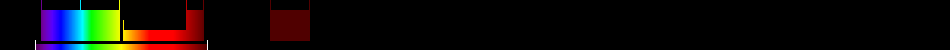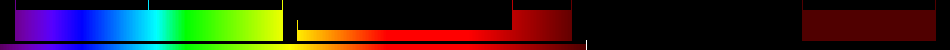Nr. Name Start WL Middle WL End WL Sp.Rg. Spat. Res. Comment
1 Pan 585 675 765 180 0.12
2 Blue 395 440 485 90 0.12
3 Green 485 530 575 90 0.12
4 Red 730 750 770 40 0.12
5 Infrared 925 970 1015 90 0.12

## Indices

Nr. Name Specific Formula Calculated Comment
1 Adjusted transformed soil-adjusted VI $1.22\frac{{\mathrm{Infrared}}-1.22{\mathrm{Red}}-0.03}{1.22{\mathrm{Infrared}}+{\mathrm{Red}}-1.22·0.03+0.08\left(1+{1.22}^{2}\right)}$ Automatic
2 Atmospherically Resistant Vegetation Index $\frac{{\mathrm{Infrared}}-{\mathrm{Red}}-{y}\left({\mathrm{Red}}-{\mathrm{Blue}}\right)}{{\mathrm{Infrared}}+{\mathrm{Red}}-{y}\left({\mathrm{Red}}-{\mathrm{Blue}}\right)}$ Automatic
3 Atmospherically Resistant Vegetation Index 2 $-0.18+1.17\left(\frac{{\mathrm{Infrared}}-{\mathrm{Red}}}{{\mathrm{Infrared}}+{\mathrm{Red}}}\right)$ Automatic
4 Blue-wide dynamic range vegetation index $\frac{0.1{\mathrm{Infrared}}-{\mathrm{Blue}}}{0.1{\mathrm{Infrared}}+{\mathrm{Blue}}}$ Automatic
5 Canopy Chlorophyll Content Index $\frac{\frac{{\mathrm{Infrared}}-{\mathrm{Red}}}{{\mathrm{Infrared}}+{\mathrm{Red}}}}{\frac{{\mathrm{Infrared}}-{\mathrm{Red}}}{{\mathrm{Infrared}}+{\mathrm{Red}}}}$ Automatic
6 Chlorophyll Green ${\left(\frac{{\mathrm{Red}}}{{\mathrm{Green}}}\right)}^{\left(-1\right)}$ Automatic
7 Chlorophyll Index Green $\frac{{\mathrm{Infrared}}}{{\mathrm{Green}}}-1$ Automatic
8 Chlorophyll IndexRedEdge $\frac{{\mathrm{Infrared}}}{{\mathrm{Red}}}-1$ Automatic
9 Chlorophyll vegetation index ${\mathrm{Infrared}}\frac{{\mathrm{Red}}}{{{\mathrm{Green}}}^{2}}$ Automatic
10 Coloration Index $\frac{{\mathrm{Red}}-{\mathrm{Blue}}}{{\mathrm{Red}}}$ Automatic
11 Corrected Transformed Vegetation Index $\frac{\left(\frac{{\mathrm{Red}}-{\mathrm{Green}}}{{\mathrm{Red}}+{\mathrm{Green}}}\right)+0,5}{|\left(\frac{{\mathrm{Red}}-{\mathrm{Green}}}{{\mathrm{Red}}+{\mathrm{Green}}}\right)+0,5|}·\sqrt{|\left(\left(\frac{{\mathrm{Red}}-{\mathrm{Green}}}{{\mathrm{Red}}+{\mathrm{Green}}}\right)\right)+0,5|}$ Automatic
12 Difference NIR/Green ${\mathrm{Infrared}}-{\mathrm{Green}}$ Automatic
13 Enhanced Vegetation Index $2.5\frac{{\mathrm{Infrared}}-{\mathrm{Red}}}{\left({\mathrm{Infrared}}+6{\mathrm{Red}}-7.5{\mathrm{Blue}}\right)+1}$ Automatic
14 Enhanced Vegetation Index 2 $2.4\frac{{\mathrm{Infrared}}-{\mathrm{Red}}}{{\mathrm{Infrared}}+{\mathrm{Red}}+1}$ Automatic
15 Enhanced Vegetation Index 2 $2.5\frac{{\mathrm{Infrared}}-{\mathrm{Red}}}{{\mathrm{Infrared}}+2.4{\mathrm{Red}}+1}$ Automatic
16 Global Environment Monitoring Index $\left(\frac{2\left({{\mathrm{Infrared}}}^{2}-{{\mathrm{Red}}}^{2}\right)+1.5{\mathrm{Infrared}}+0.5{\mathrm{Red}}}{{\mathrm{Infrared}}+{\mathrm{Red}}+0.5}\left(1-0.25\frac{2\left({{\mathrm{Infrared}}}^{2}-{{\mathrm{Red}}}^{2}\right)+1.5{\mathrm{Infrared}}+0.5{\mathrm{Red}}}{{\mathrm{Infrared}}+{\mathrm{Red}}+0.5}\right)-\frac{{\mathrm{Red}}-0.125}{1-{\mathrm{Red}}}\right)$ Automatic
17 Green atmospherically resistant vegetation index $\frac{{\mathrm{Infrared}}-\left({\mathrm{Green}}-\left({\mathrm{Blue}}-{\mathrm{Red}}\right)\right)}{{\mathrm{Infrared}}-\left({\mathrm{Green}}+\left({\mathrm{Blue}}-{\mathrm{Red}}\right)\right)}$ Automatic
18 Green leaf index $\frac{2{\mathrm{Green}}-{\mathrm{Red}}-{\mathrm{Blue}}}{2{\mathrm{Green}}+{\mathrm{Red}}+{\mathrm{Blue}}}$ Automatic
19 Green Normalized Difference Vegetation Index $\frac{{\mathrm{Infrared}}-{\mathrm{Green}}}{{\mathrm{Infrared}}+{\mathrm{Green}}}$ Automatic
20 Green Optimized Soil Adjusted Vegetation Index $\frac{{\mathrm{Infrared}}-{\mathrm{Green}}}{{\mathrm{Infrared}}+{\mathrm{Green}}+{Y}}$ Automatic
21 Green Soil Adjusted Vegetation Index $\frac{{\mathrm{Infrared}}-{\mathrm{Green}}}{{\mathrm{Infrared}}+{\mathrm{Green}}+{L}}\left(1+{L}\right)$ Automatic
22 Green-Blue NDVI $\frac{{\mathrm{Infrared}}-\left({\mathrm{Green}}+{\mathrm{Blue}}\right)}{{\mathrm{Infrared}}+\left({\mathrm{Green}}+{\mathrm{Blue}}\right)}$ Automatic
23 Green-Red NDVI $\frac{{\mathrm{Infrared}}-\left({\mathrm{Green}}+{\mathrm{Red}}\right)}{{\mathrm{Infrared}}+\left({\mathrm{Green}}+{\mathrm{Red}}\right)}$ Automatic
24 Hue $\mathrm{arctan}\left(\frac{2{\mathrm{Red}}-{\mathrm{Green}}-{\mathrm{Blue}}}{30.5}\left({\mathrm{Green}}-{\mathrm{Blue}}\right)\right)$ Automatic
25 Ideal vegetation index $\frac{{\mathrm{Infrared}}-{b}}{{a}·{\mathrm{Red}}}$ Automatic
26 Infrared percentage vegetation index $\frac{\frac{{\mathrm{Infrared}}}{{\mathrm{Infrared}}+{\mathrm{Red}}}}{2}\left(\left(\frac{{\mathrm{Red}}-{\mathrm{Green}}}{{\mathrm{Red}}+{\mathrm{Green}}}\right)+1\right)$ Automatic
27 Intensity $\left(\frac{1}{30.5}\right)\left({\mathrm{Red}}+{\mathrm{Green}}+{\mathrm{Blue}}\right)$ Automatic
28 Inverse reflectance 550 ${{\mathrm{Green}}}^{\left(-1\right)}$ Automatic
29 Log Ratio $\mathrm{log}\left(\frac{{\mathrm{Infrared}}}{{\mathrm{Red}}}\right)$ Automatic
30 Modified Simple Ratio NIR/RED $\frac{\left(\frac{{\mathrm{Infrared}}}{{\mathrm{Red}}}\right)-1}{\sqrt{\left(\frac{{\mathrm{Infrared}}}{{\mathrm{Red}}}\right)+1}}$ Automatic
31 Modified Soil Adjusted Vegetation Index $\frac{2{\mathrm{Infrared}}+1-\sqrt{{\left(2{\mathrm{Infrared}}+1\right)}^{2}-8\left({\mathrm{Infrared}}-{\mathrm{Red}}\right)}}{2}$ Automatic
32 Nonlinear vegetation index $\frac{{{\mathrm{Infrared}}}^{2}-{\mathrm{Red}}}{{{\mathrm{Infrared}}}^{2}+{\mathrm{Red}}}$ Automatic
33 Norm G $\frac{{\mathrm{Green}}}{{\mathrm{Infrared}}+{\mathrm{Red}}+{\mathrm{Green}}}$ Automatic
34 Norm NIR $\frac{{\mathrm{Infrared}}}{{\mathrm{Infrared}}+{\mathrm{Red}}+{\mathrm{Green}}}$ Automatic
35 Norm R $\frac{{\mathrm{Red}}}{{\mathrm{Infrared}}+{\mathrm{Red}}+{\mathrm{Green}}}$ Automatic
36 Normalized Difference 550/450 $\frac{{\mathrm{Green}}-{\mathrm{Blue}}}{{\mathrm{Green}}+{\mathrm{Blue}}}$ Automatic
37 Normalized Difference 750/550 $\frac{{\mathrm{Red}}-{\mathrm{Green}}}{{\mathrm{Red}}+{\mathrm{Green}}}$ Automatic
38 Normalized Difference Green/Red $\frac{{\mathrm{Green}}-{\mathrm{Red}}}{{\mathrm{Green}}+{\mathrm{Red}}}$ Automatic
39 Normalized Difference NIR/Blue $\frac{{\mathrm{Infrared}}-{\mathrm{Blue}}}{{\mathrm{Infrared}}+{\mathrm{Blue}}}$ Automatic
40 Normalized Difference NIR/Green $\frac{{\mathrm{Infrared}}-{\mathrm{Green}}}{{\mathrm{Infrared}}+{\mathrm{Green}}}$ Automatic
41 Normalized Difference NIR/Rededge $\frac{{\mathrm{Infrared}}-{\mathrm{Red}}}{{\mathrm{Infrared}}+{\mathrm{Red}}}$ Automatic
42 Normalized Difference Red/Green $\frac{{\mathrm{Red}}-{\mathrm{Green}}}{{\mathrm{Red}}+{\mathrm{Green}}}$ Automatic
43 Pan NDVI $\frac{{\mathrm{Infrared}}-\left({\mathrm{Green}}+{\mathrm{Red}}+{\mathrm{Blue}}\right)}{{\mathrm{Infrared}}+\left({\mathrm{Green}}+{\mathrm{Red}}+{\mathrm{Blue}}\right)}$ Automatic
44 Ratio Analysis of Reflectance Spectra C1 $\frac{\frac{{\mathrm{Red}}}{{\mathrm{Green}}}}{\frac{{\mathrm{r760}}}{{\mathrm{r500}}}}$ Automatic
45 Ratio Analysis of Reflectance Spectra C2 $\frac{\frac{{\mathrm{Red}}}{{\mathrm{Blue}}}}{\frac{{\mathrm{r760}}}{{\mathrm{r470}}}}$ Automatic
46 Red-Blue NDVI $\frac{{\mathrm{Infrared}}-\left({\mathrm{Red}}+{\mathrm{Blue}}\right)}{{\mathrm{Infrared}}+\left({\mathrm{Red}}+{\mathrm{Blue}}\right)}$ Automatic
47 Shape Index $\frac{2{\mathrm{Red}}-{\mathrm{Green}}-{\mathrm{Blue}}}{{\mathrm{Green}}-{\mathrm{Blue}}}$ Automatic
48 Simple Ratio 440/740 $\frac{{\mathrm{Blue}}}{{\mathrm{Red}}}$ Automatic
49 Simple Ratio 450/550 $\frac{{\mathrm{Blue}}}{{\mathrm{Green}}}$ Automatic
50 Simple Ratio 520/420 $\frac{{\mathrm{Green}}}{{\mathrm{Blue}}}$ Automatic
51 Simple Ratio 520/760 $\frac{{\mathrm{Green}}}{{\mathrm{Red}}}$ Automatic
52 Simple Ratio 542/750 $\frac{{\mathrm{Green}}}{{\mathrm{Red}}}$ Automatic
53 Simple Ratio 550/420 $\frac{{\mathrm{Green}}}{{\mathrm{Blue}}}$ Automatic
54 Simple Ratio 550/760 $\frac{{\mathrm{Green}}}{{\mathrm{Red}}}$ Automatic
55 Simple Ratio 556/750 $\frac{{\mathrm{Green}}}{{\mathrm{Red}}}$ Automatic
56 Simple Ratio 750/550 $\frac{{\mathrm{Red}}}{{\mathrm{Green}}}$ Automatic
57 Simple Ratio 750/555 $\frac{{\mathrm{Red}}}{{\mathrm{Green}}}$ Automatic
58 Simple Ratio 760/500 $\frac{{\mathrm{Red}}}{{\mathrm{Green}}}$ Automatic
59 Simple Ratio NIR/G $\frac{{\mathrm{Infrared}}}{{\mathrm{Green}}}$ Automatic
60 Simple Ratio NIR/RED $\frac{{\mathrm{Infrared}}}{{\mathrm{Red}}}$ Automatic
61 Simple Ratio NIR/Rededge $\frac{{\mathrm{Infrared}}}{{\mathrm{Red}}}$ Automatic
62 Simple Ratio Red/Blue $\frac{{\mathrm{Red}}}{{\mathrm{Blue}}}$ Automatic
63 Simple Ratio Red/Green $\frac{{\mathrm{Red}}}{{\mathrm{Green}}}$ Automatic
64 Simple Ratio Red/NIR $\frac{{\mathrm{Red}}}{{\mathrm{Infrared}}}$ Automatic
65 Single Band 430 ${\mathrm{Blue}}$ Automatic
66 Single Band 460 ${\mathrm{Blue}}$ Automatic
67 Single Band 470 ${\mathrm{Blue}}$ Automatic
68 Single Band 495 ${\mathrm{Green}}$ Automatic
69 Single Band 550 ${\mathrm{Green}}$ Automatic
70 Single Band 555 ${\mathrm{Green}}$ Automatic
71 Single Band 735 ${\mathrm{Red}}$ Automatic
72 Single Band 930 ${\mathrm{Infrared}}$ Automatic
73 Single Band 970 ${\mathrm{Infrared}}$ Automatic
74 Single Band 990 ${\mathrm{Infrared}}$ Automatic
75 Soil and Atmospherically Resistant Vegetation Index 2 $2,5\frac{{\mathrm{Infrared}}-{\mathrm{Red}}}{1+{\mathrm{Infrared}}+6{\mathrm{Red}}-7,5{\mathrm{Blue}}}$ Automatic
76 Soil-adjusted vegetation index 2 $\frac{{\mathrm{Infrared}}}{{\mathrm{Red}}+\frac{{b}}{{a}}}$ Automatic
77 SQRT(IR/R) $\sqrt{\frac{{\mathrm{Infrared}}}{{\mathrm{Red}}}}$ Automatic
78 Transformed NDVI $\sqrt{\frac{{\mathrm{Infrared}}-{\mathrm{Red}}}{{\mathrm{Infrared}}+{\mathrm{Red}}}+0.5}$ Automatic
79 Transformed Soil Adjusted Vegetation Index $\frac{{B}\left({\mathrm{Infrared}}-{B}·{\mathrm{Red}}-{A}\right)}{{\mathrm{Red}}+{B}\left({\mathrm{Infrared}}-{A}\right)+{X}\left(1+{{B}}^{2}\right)}$ Automatic
80 Transformed Soil Adjusted Vegetation Index 2 $\frac{{a}·{\mathrm{Infrared}}-{a}·{\mathrm{Red}}-{b}}{{\mathrm{Red}}+{a}·{\mathrm{Infrared}}-{a}·{b}}$ Automatic
81 Transformed Vegetation Index $\sqrt{\left(\left(\frac{{\mathrm{Red}}-{\mathrm{Green}}}{{\mathrm{Red}}+{\mathrm{Green}}}\right)\right)+0,5}$ Automatic
82 Weighted Difference Vegetation Index ${\mathrm{Infrared}}-{a}·{\mathrm{Red}}$ Automatic
83 Wide Dynamic Range Vegetation Index $\frac{0.1{\mathrm{Infrared}}-{\mathrm{Red}}}{0.1{\mathrm{Infrared}}+{\mathrm{Red}}}$ Automatic

## Applications

Nr. Name
1 Agriculture
2 Agriculture - Crop parameters
3 Agriculture - Precision Crop Management
4 Geology
5 Hyperspectral remote sensing - Red-edge position
6 Metal
7 Metal - Iron
8 Oil
9 Soil
10 Vegetation
11 Vegetation - Biomass
12 Vegetation - Cellulose
13 Vegetation - Chlorophyll
14 Vegetation - LAI
15 Vegetation - Lignin
16 Vegetation - Starch
17 Vegetation - Vitality
18 Vegetation - Water

Export bibliographic references as: BibTeXRIS (Endnote)CSV

## Visualisation of Indices

Sensor:Indices: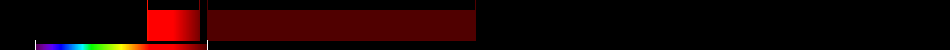Atmospherically Resistant Vegetation Index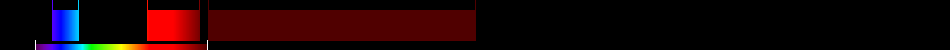Atmospherically Resistant Vegetation Index 2Blue-wide dynamic range vegetation index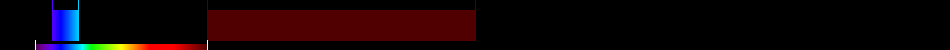Canopy Chlorophyll Content Index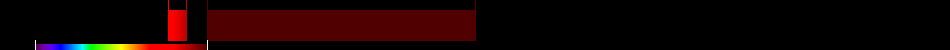Chlorophyll Green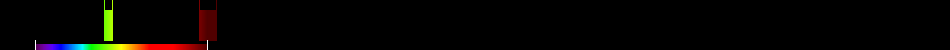Chlorophyll Index Green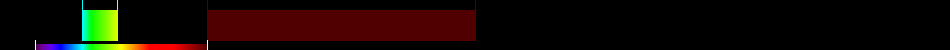Chlorophyll IndexRedEdgeChlorophyll vegetation index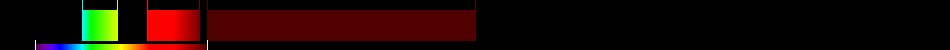Coloration Index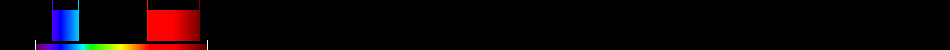Corrected Transformed Vegetation Index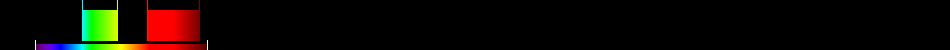Difference NIR/GreenEnhanced Vegetation Index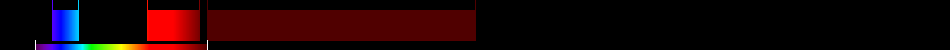Enhanced Vegetation Index 2Enhanced Vegetation Index 2Global Environment Monitoring IndexGreen atmospherically resistant vegetation index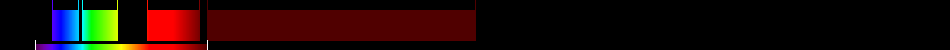Green leaf index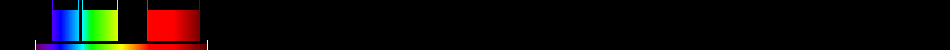Green Normalized Difference Vegetation Index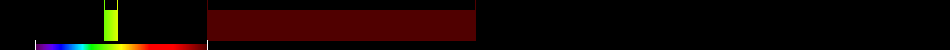Green Optimized Soil Adjusted Vegetation IndexGreen-Blue NDVI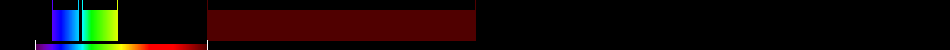Green-Red NDVIHueIdeal vegetation indexInfrared percentage vegetation indexIntensityInverse reflectance 550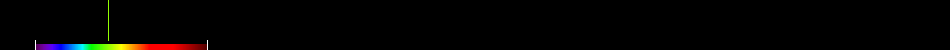Log RatioModified Simple Ratio NIR/REDNonlinear vegetation indexNorm GNorm NIRNorm RNormalized Difference 550/450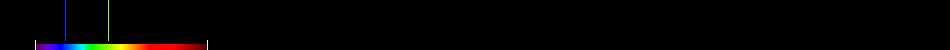Normalized Difference 750/550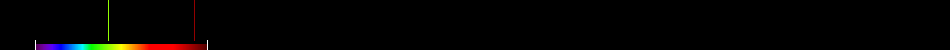Normalized Difference Green/RedNormalized Difference NIR/BlueNormalized Difference NIR/GreenNormalized Difference NIR/RededgeNormalized Difference Red/GreenPan NDVIRatio Analysis of Reflectance Spectra C1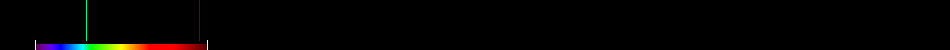Ratio Analysis of Reflectance Spectra C2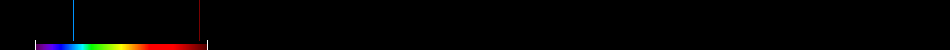Red-Blue NDVIShape IndexSimple Ratio 440/740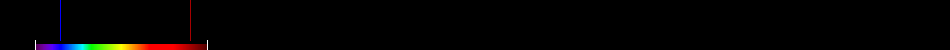Simple Ratio 450/550Simple Ratio 520/420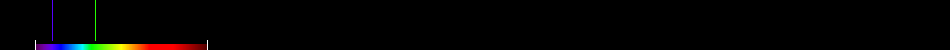Simple Ratio 520/760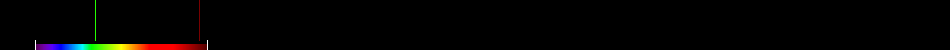Simple Ratio 542/750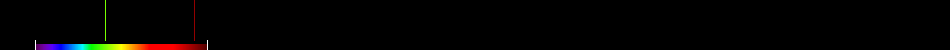Simple Ratio 550/420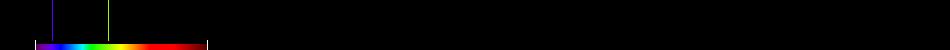Simple Ratio 550/760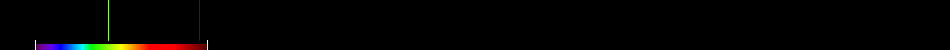Simple Ratio 556/750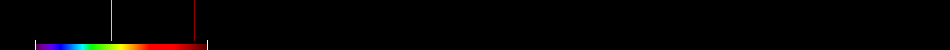Simple Ratio 750/550Simple Ratio 750/555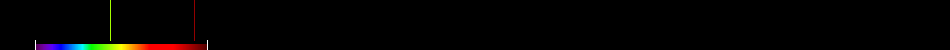Simple Ratio 760/500Simple Ratio NIR/GSimple Ratio NIR/REDSimple Ratio NIR/RededgeSimple Ratio Red/BlueSimple Ratio Red/GreenSimple Ratio Red/NIRSingle Band 430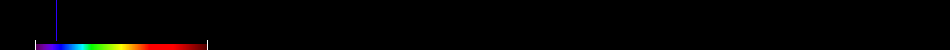Single Band 460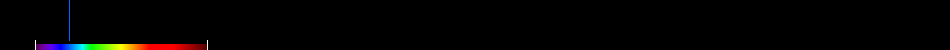Single Band 470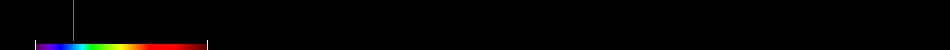Single Band 495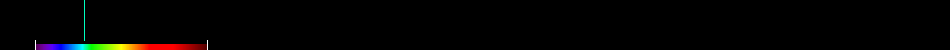Single Band 550Single Band 555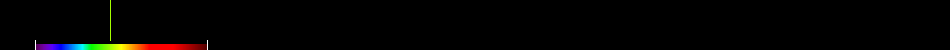Single Band 735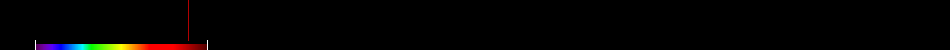Single Band 930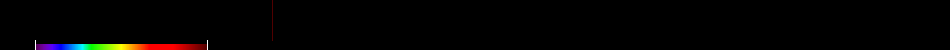Single Band 970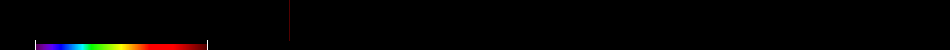Single Band 990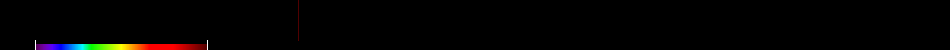Soil and Atmospherically Resistant Vegetation Index 2SQRT(IR/R)Transformed NDVI